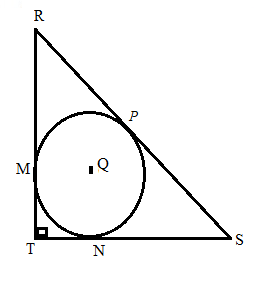Chapter 6.3, Problem 36EElementary Geometry For College St...

7th Edition
Alexander + 2 others
ISBN: 9781337614085

Solutions

Chapter
SectionElementary Geometry For College St...

7th Edition
Alexander + 2 others
ISBN: 9781337614085
Textbook Problem

Given: ⊙ Q is inscribed in isosceles right Δ R S T . The perimeter of Δ R S T is 8 + 4 2 Find: TMTo determine

To find:

To find TM.

Explanation

Given that, Q is inscribed in isosceles right ΔRST. The perimeter of ΔRST is 8+42.

The tangents segments from an external point are congruent. Therefore,

RP=RM=xSP=SN=yTN=TM=z

The diagrammatic representation is given below,

Perimeter of the ΔRST is 8+42.

That is RT+TS+RS=8+42

Given that ΔRST is an isosceles triangle. Therefore, RT=TS.

Then the above equation become the following,

RT+TS+RS=8+42RT+RT+RS=8+422RT+RS=8+42.........(1)

By using the Pythagorean theorem to get the following,

RT2+TS2=RS2RT2+RT2=RS22RT2=RS2. Since RT=TS

Taking square root on both sides to get the following,

RS=RT2...........(2)

Substitute equation (2) in (1) to get the following,

2RT+RT2=8+42RT(2+2)=8+42RT=4(2+2)(2+2)RT=4

Also, know that RT=TS=4

By using the Pythagorean theorem to get the following,

RT2+TS2=RS242+42=RS216+16=RS2RS=32RS=42.

The value of RT=4,TS=4,andRS=42

We know that,

RS=RP+PS=x+yTS=TN+NS=z+yRT=RM+MT=x+z

Substitute the value of RT=4,TS=4,andRS=42 in the above equation to get the following,

x+y=42

Still sussing out bartleby?

Check out a sample textbook solution.

See a sample solution

The Solution to Your Study Problems

Bartleby provides explanations to thousands of textbook problems written by our experts, many with advanced degrees!

Get Started

Expand each expression in Exercises 122. (4y2)y

Finite Mathematics and Applied Calculus (MindTap Course List)

Area In Exercises 73-76, find the area or the given region. y=3x+2x2+9

Calculus: Early Transcendental Functions (MindTap Course List)

The x-coordinate of the center of mass of the region bounded by , x = 1, x = 2, y = 0 is: ln 2 1 2 ln 2

Study Guide for Stewart's Single Variable Calculus: Early Transcendentals, 8th

Using a binomial series, the Maclaurin series for is:

Study Guide for Stewart's Multivariable Calculus, 8th